Qur’an Tools is not optimised for narrow screens

If you select List Lemmata from the Browse menu (where it can be found under Word Lists), Qur’an Tools will display a list of the 4,817 different lemmata that occur in the qur’anic text.

If you are unfamiliar with the term lemmata, it is the plural of the term lemma. The lemma is the word that stands at the head of an entry in a dictionary; for example, here is the lemma kātib defined in Lane’s Arabic-English Lexicon: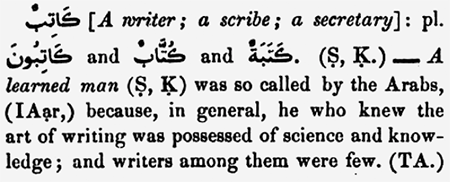Each Arabic root in the Qur’an will usually be expressed in multiple lemmata. For example, here are the  seven lemmata based on the root ktb that can be found in the qur’anic text:The List Lemmata tool allows you to easily explore all the lemmata in the Qur’an; it looks like this: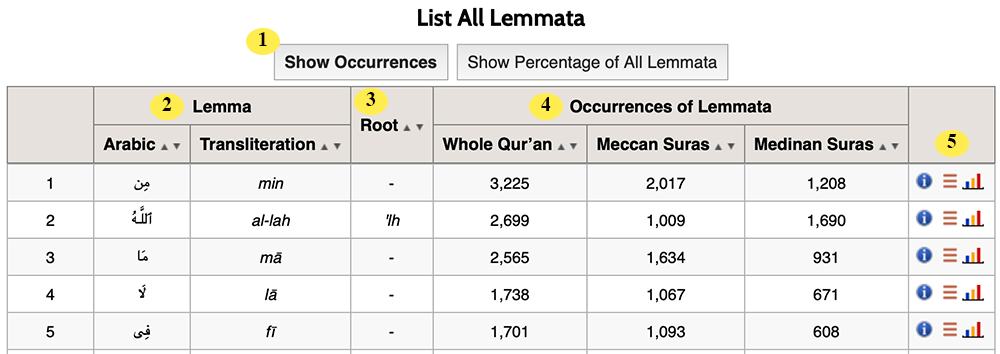1 Show Occurrences or Show Percentages. You can choose whether the lemmata list counts the occurrences of each lemma in the Qur'an (for example, min occurs 3,225 times) or whether you’d prefer to see the percentage of lemmata that each lemma makes up — for example, click "Show Percentage of All Lemmata" and you’ll discover that min makes up 4.323% of all lemmata in the Qur’an. 2 Lemma. For each lemma in the list, Qur’an Tools shows you both its Arabic and its transliterated version. (If you’d like to sort the table by lemma, just use the arrows at the top of the column — indeed, you can sort the table by any column you like this way). 3 Root. This column shows the root that each lemma is based on (if the lemma is based on a root — for example, min is a particle-preposition, so it has no root. If there is a root listed here, you may click it on it to run a search for where it occurs in the Qur’an. 4 Occurrences. For each lemma, Qur’an Tools shows you how often that lemma occurs in the whole Qur’an, in Meccan suras, and in Medinan suras. Click on any number in these columns and Qur’an Tools will show you the verses where the root occurs. 5 Action Icons. Click the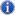icon to view additional information about the root underlying this lemma (if the lemma has a root); click the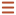icon to exhaustively list all the occurrences in the Qur’an of this lemma; or click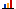to display a chart of this lemma’s occurrences in the Qur’an (or simply point at theicon and wait a few seconds to see a mini-chart, like the example below).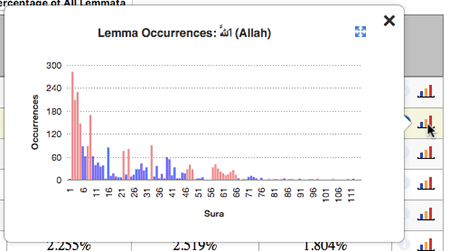At the bottom of the root list, you’ll find a page navigator, as displaying the entire list of lemmata on one screen slows some web browsers to a crawl.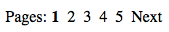Just click on a number to move between pages.